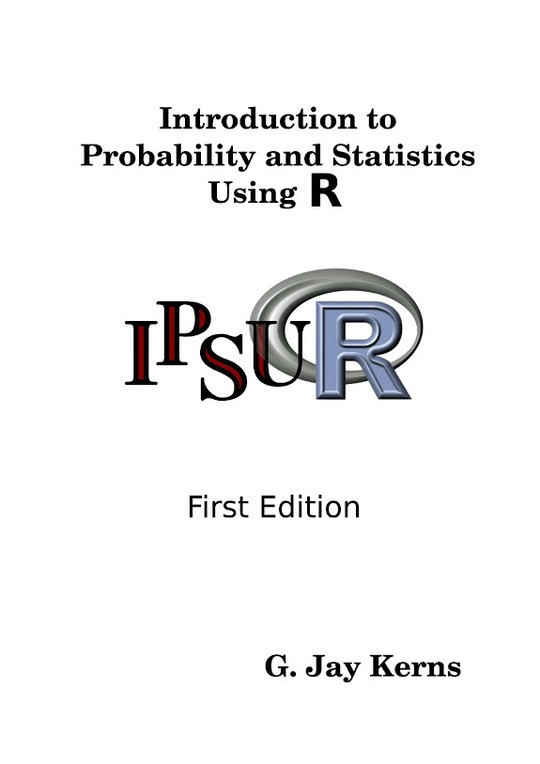G. Jay Kerns
Computers & Technology
Introduction to Probability and Statistics Using R
Free
Description
Contents
Reviews

This is a textbook for an undergraduate course in probability and statistics. The approximate prerequisites are two or three... More > semesters of calculus and some linear algebra. Students attending the class include mathematics, engineering, and computer science majors.

Language
English
ISBN
978-0-557-24979-4
Contents
Preface
List of Figures
List of Tables
1 An Introduction to Probability and Statistics
1.1 Probability
1.2 Statistics
Chapter Exercises
2 An Introduction to R
2.2 Communicating with R
2.3 Basic R Operations and Concepts
2.4 Getting Help
2.5 External Resources
2.6 Other Tips
Chapter Exercises
3 Data Description
3.1 Types of Data
3.2 Features of Data Distributions
3.3 Descriptive Statistics
3.4 Exploratory Data Analysis
3.5 Multivariate Data and Data Frames
3.6 Comparing Populations
Chapter Exercises
4 Probability
4.1 Sample Spaces
4.2 Events
4.3 Model Assignment
4.4 Properties of Probability
4.5 Counting Methods
4.6 Conditional Probability
4.7 Independent Events
4.8 Bayes' Rule
4.9 Random Variables
Chapter Exercises
5 Discrete Distributions
5.1 Discrete Random Variables
5.2 The Discrete Uniform Distribution
5.3 The Binomial Distribution
5.4 Expectation and Moment Generating Functions
5.5 The Empirical Distribution
5.6 Other Discrete Distributions
5.7 Functions of Discrete Random Variables
Chapter Exercises
6 Continuous Distributions
6.1 Continuous Random Variables
6.2 The Continuous Uniform Distribution
6.3 The Normal Distribution
6.4 Functions of Continuous Random Variables
6.5 Other Continuous Distributions
Chapter Exercises
7 Multivariate Distributions
7.1 Joint and Marginal Probability Distributions
7.2 Joint and Marginal Expectation
7.3 Conditional Distributions
7.4 Independent Random Variables
7.5 Exchangeable Random Variables
7.6 The Bivariate Normal Distribution
7.7 Bivariate Transformations of Random Variables
7.8 Remarks for the Multivariate Case
7.9 The Multinomial Distribution
Chapter Exercises
8 Sampling Distributions
8.1 Simple Random Samples
8.2 Sampling from a Normal Distribution
8.3 The Central Limit Theorem
8.4 Sampling Distributions of Two-Sample Statistics
8.5 Simulated Sampling Distributions
Chapter Exercises
9 Estimation
9.1 Point Estimation
9.2 Confidence Intervals for Means
9.3 Confidence Intervals for Differences of Means
9.4 Confidence Intervals for Proportions
9.5 Confidence Intervals for Variances
9.6 Fitting Distributions
9.7 Sample Size and Margin of Error
9.8 Other Topics
Chapter Exercises
10 Hypothesis Testing
10.1 Introduction
10.2 Tests for Proportions
10.3 One Sample Tests for Means and Variances
10.4 Two-Sample Tests for Means and Variances
10.5 Other Hypothesis Tests
10.6 Analysis of Variance
10.7 Sample Size and Power
Chapter Exercises
11 Simple Linear Regression
11.1 Basic Philosophy
11.2 Estimation
11.3 Model Utility and Inference
11.4 Residual Analysis
11.5 Other Diagnostic Tools
Chapter Exercises
12 Multiple Linear Regression
12.1 The Multiple Linear Regression Model
12.2 Estimation and Prediction
12.3 Model Utility and Inference
12.4 Polynomial Regression
12.5 Interaction
12.6 Qualitative Explanatory Variables
12.7 Partial F Statistic
12.8 Residual Analysis and Diagnostic Tools
Chapter Exercises
13 Resampling Methods
13.1 Introduction
13.2 Bootstrap Standard Errors
13.3 Bootstrap Confidence Intervals
13.4 Resampling in Hypothesis Tests
Chapter Exercises
14 Categorical Data Analysis
15 Nonparametric Statistics
16 Time Series
A R Session Information
C History
D Data
D.1 Data Structures
D.2 Importing Data
D.3 Creating New Data Sets
D.4 Editing Data
D.5 Exporting Data
D.6 Reshaping Data
E Mathematical Machinery
E.1 Set Algebra
E.2 Differential and Integral Calculus
E.3 Sequences and Series
E.4 The Gamma Function
E.5 Linear Algebra
E.6 Multivariable Calculus
F Writing Reports with R
F.1 What to Write
F.2 How to Write It with R
F.3 Formatting Tables
F.4 Other Formats
G Instructions for Instructors
G.1 Generating This Document
G.2 How to Use This Document
G.3 Ancillary Materials
G.4 Modifying This Document
H RcmdrTestDrive Story
Bibliography
Index
The book hasn't received reviews yet.